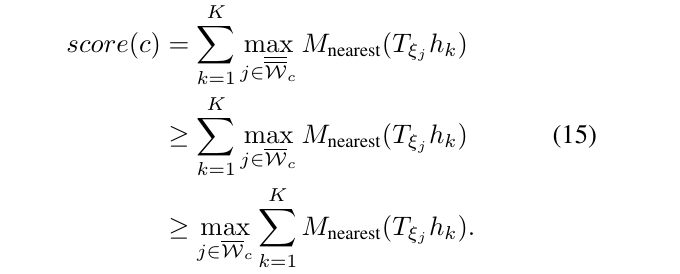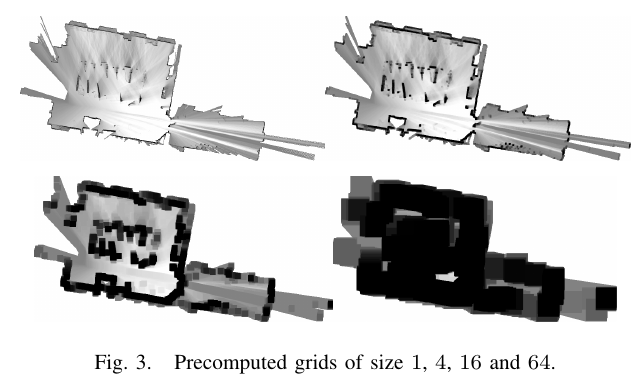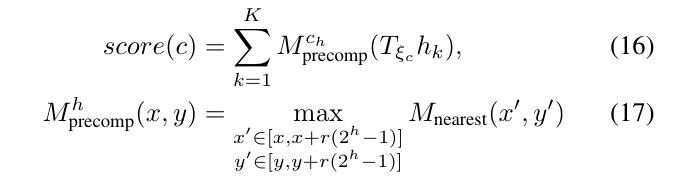# 1. Real-Time Loop Closure in 2D LIDAR SLAM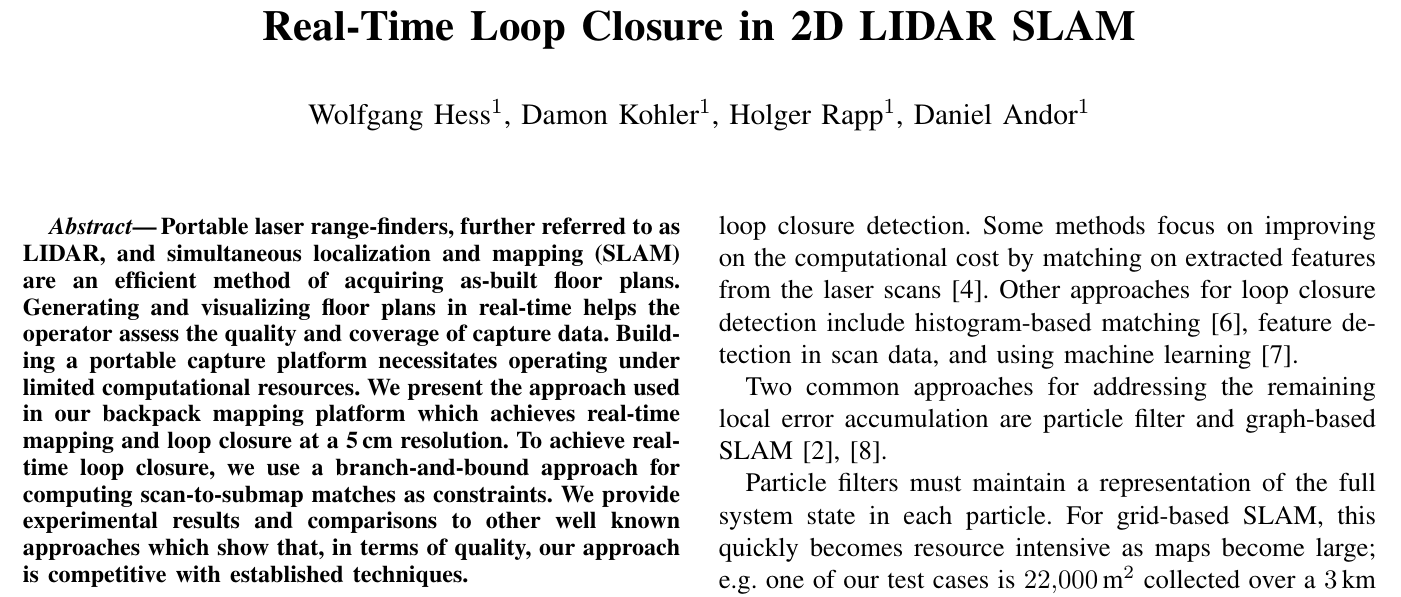# 6. 局部2D-SLAM

## 6.1. Scans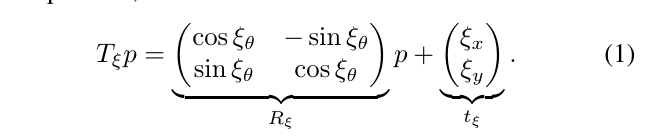## 6.2. Submaps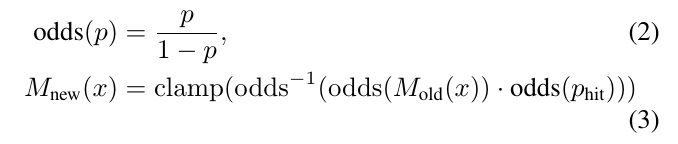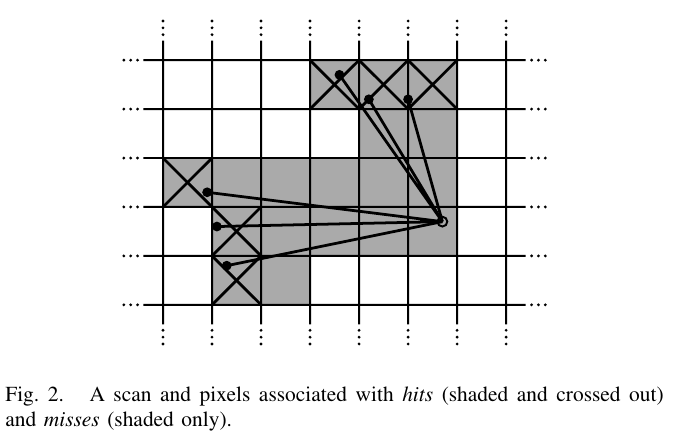## 6.3. 在 scan matching使用Ceres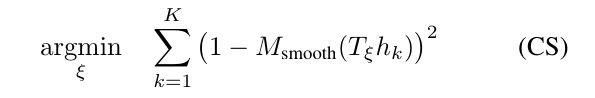# 7. 回环

## 7.1. 优化问题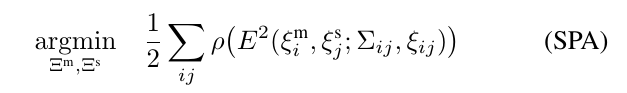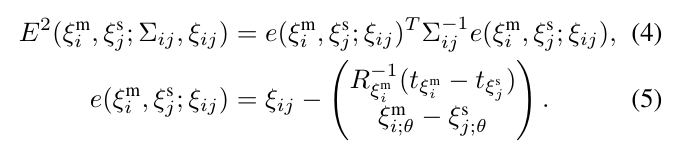## 7.2. 分枝定界的scan matching

### 7.2.1. 目标函数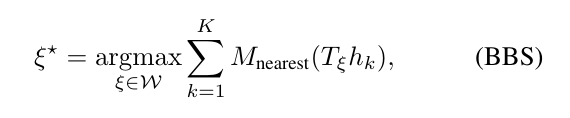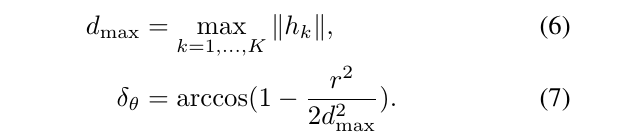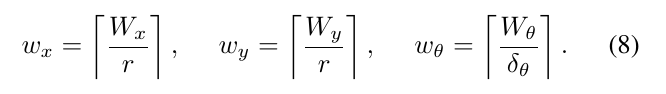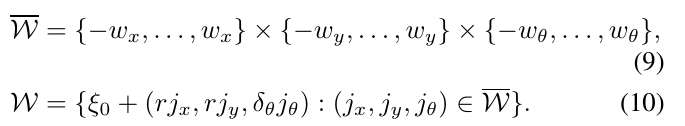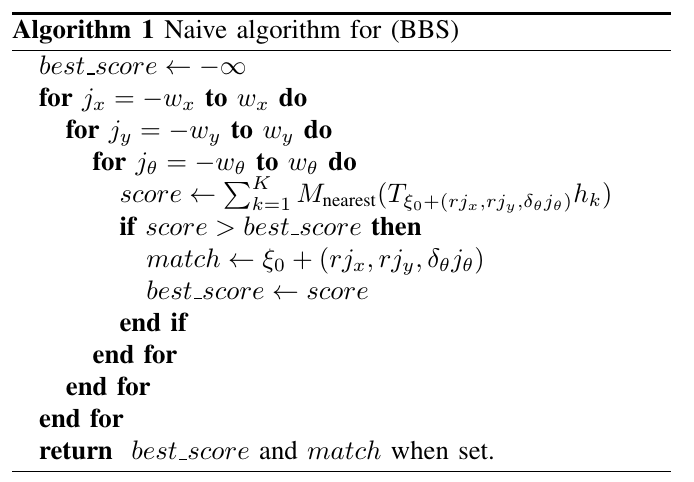### 7.2.2. 分枝定界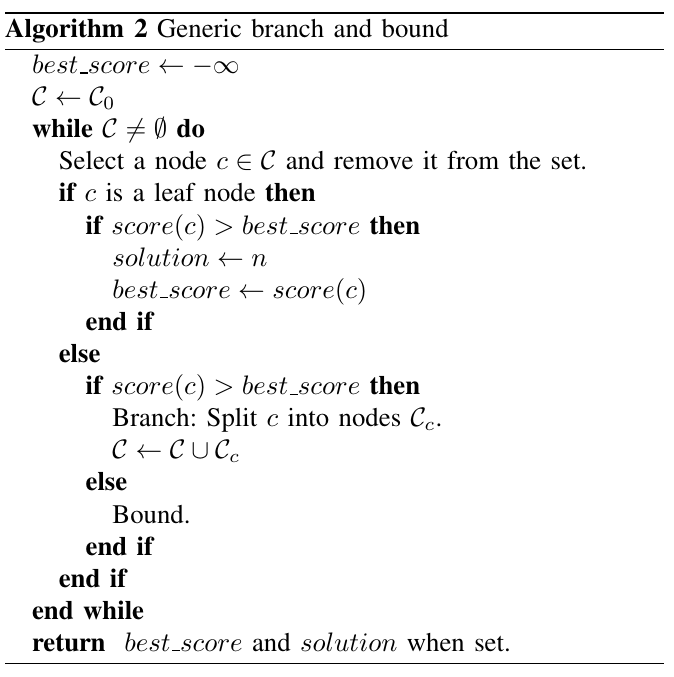• 每个节点的子节点形成其父节点的一个分区，因此它们一起表示相同的一组可能性。
• 叶节点是单节点;每一个都代表一个可行的解决方案
• 注意到算法是精确的，它提供了与朴素方法相同的解决方案，其中的前提条件是只要内部某个节点$$c$$的得分$$Score(c)$$是其所有子节点得分的上限
• 在这种情况下，当一个节点有界(上限)时，这个节点的所有子节点就不存在比已知的最好的解决方案更好的解决方案

### 7.2.3. 分枝定界树的构建

#### 7.2.3.1. 节点选择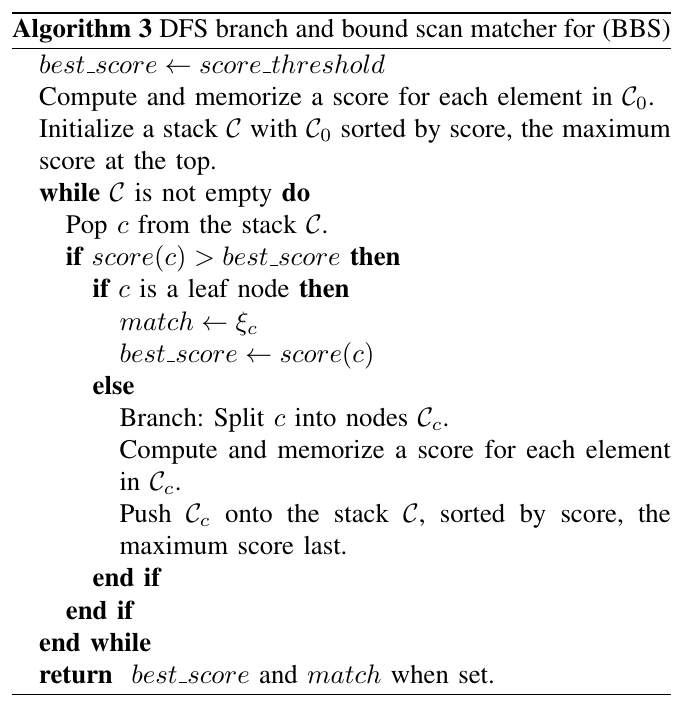• 最佳分数设置为给定的阈值
• 计算和储存集合$$C_0$$的每一个元素的得分
• 对集合$$C_0$$按得分排序，初始化堆栈，分数最高的在顶部。
• 如果堆栈$$C$$不为空，则进入遍历：
• 从堆栈$$C$$弹出顶部元素$$c$$（得分最高的）
• 如果$$c$$的得分比最佳分数高
• 同时也是一个叶子节点（表示一种可能的解决方案），就记录下此时$$c$$所代表的位姿$$\xi_c$$，最佳分数也设置为新的值。
• 否则，若元素$$c$$不是一个叶子节点，即还没有展开到最底层，那么对元素$$c$$进一步展开，得到元素$$c$$的子集$$C_c$$，计算和储存元素$$c$$的子集$$C_c$$里面每一个元素的得分，最后将$$C_c$$推入堆栈$$C$$，按得分排序，最大分数在最顶部。
• 遍历堆栈$$C$$的所有元素之后，得到最佳分数和对应的位姿。

#### 7.2.3.2. 分支法则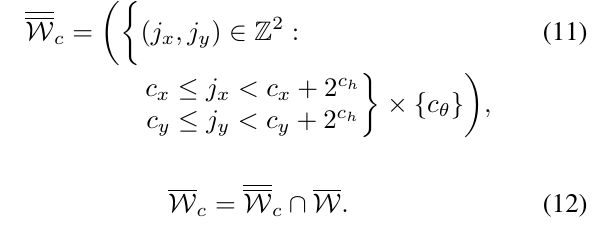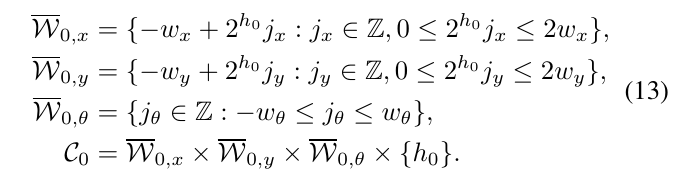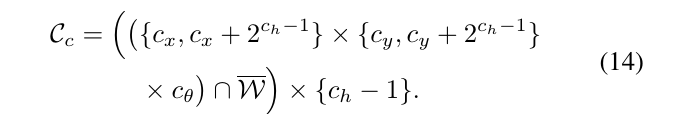#### 7.2.3.3. 计算上界